# Tidy Data Transformations

#### Package Dependencies

The core packages for tidy data transformations are listed below:

The dplyr package is by far the most important of the packages in the “tidyverse” for data transformation and manipulation.1  Verb-based functions are one of the advantages of the package.  The syntax is much easier to use when compared to the cryptic syntax of base R.

#### Example Data

Basic data transformations will be explored using the nycflights13::flights dataset supplied by the US Bureau of Transportation Statistics. The data contains all 336,776 flights that departed from New York City in 2013.  The data can be obtained as follows:

#### Subset Observations (Rows)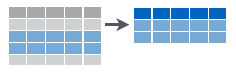The filter() function is used to subset the rows in a data.frame. The first argument is the tibble data (or data frame).  The subsequent arguments are logical expressions that refer to variables within the data.frame, selecting rows where the logicals are TRUE.

There are a number of functions to subset observations:

FunctionDescription
filter()Extract rows that meet logical criteria.
filter(iris, Sepal.Length > 7)
distinct()Remove duplicate rows
distinct(iris)
sample_frac()Randomly select a fraction of the rows
sample_frac(iris, 0.5, replace = TRUE)
sample_n()Randomly select n rows
sample_n(iris, 10, replace = TRUE)
slice()select rows by index position
slice(iris, 10:15)
top_n()Select and order the top n entries (by group for grouped data)
top_n(storms, 2, date)

#### Subset Variables (Columns)Typically, only a few columns of a large dataset are required for analysis.  The select() function narrows the variables to a useful subset and avoids the use of numeric variable positions when indexing:

Several helper functions exist for use within the select() function:

FunctionDescription
contains()Select columns whose name contains a character string
select(iris, contains("."))
ends_with()Select columns whose name ends with a character string.
select(iris, ends_with("Length"))
everything()Select every column.
select(iris, everything())
matches()Select columns whose name matches a regular expression.
select(iris, matches(".t."))
num_range()Select columns named x1, x2, x3, x4, x5.
select(iris, num_range("x", 1:5))
one_of()Select columns whose names are in a group of names.
select(iris, one_of(c("Species", "Genus")))
starts_with()Select columns whose name starts with a character string.
select(iris, starts_with("Sepal"))
x:yThe colon is used to select all columns between named columns
select(iris, Sepal.Length:Petal.Width)
-zThe minus sign is used to select all columns except named columns
select(iris, -Species)

The pull() function extracts a column data, but returns a vector.  This can be very helpful in many cases when tibbles are not desirable. For example:

#### Summarize Data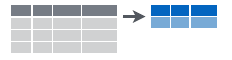The summarise() function collapses data variables to a single row summary statistic:

summarise() uses summary functions, be it base, package or custom functions.  Some standard summary functions are listed below:

FunctionDescription
first()First value of a vector
last()Last value of a vector
nth()Nth value of a vector
n()Number of values in a vector
n_distinct()Number of distinct values in a vector
IQR()Interquartile range of the vector values
min()Minimum value in a vector
max()Maximumm value of a vector
mean()Mean value of a vector
var()Variance of a vector
sd()Standard deviation of a vector

#### Group Data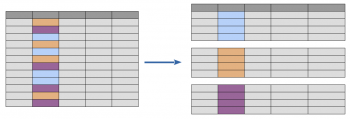Perhaps the most significant data transformation is the ability to take a large dataset and to create sub-groups on which distinct data analysis is conducted.  In the following example, all planes leaving New York are grouped by destination, the summary number of planes and flights for each destinations is calculated and then the results are ordered by the highest number of flights.  The result for 105 destinations is obtained using the pipe operator and in the absence of loops:

In the next example, the dataset is split into individual planes and for each plane a set of summary stats is calculated, including the number of flights, the average distance traveled and the observed arrival delay. Results are filtered to focus on planes with at least 20 flights and traveling less than 2,000 miles.  The output covers almost 3,000 airplanes and is presented in a plot using the ggplot2 package.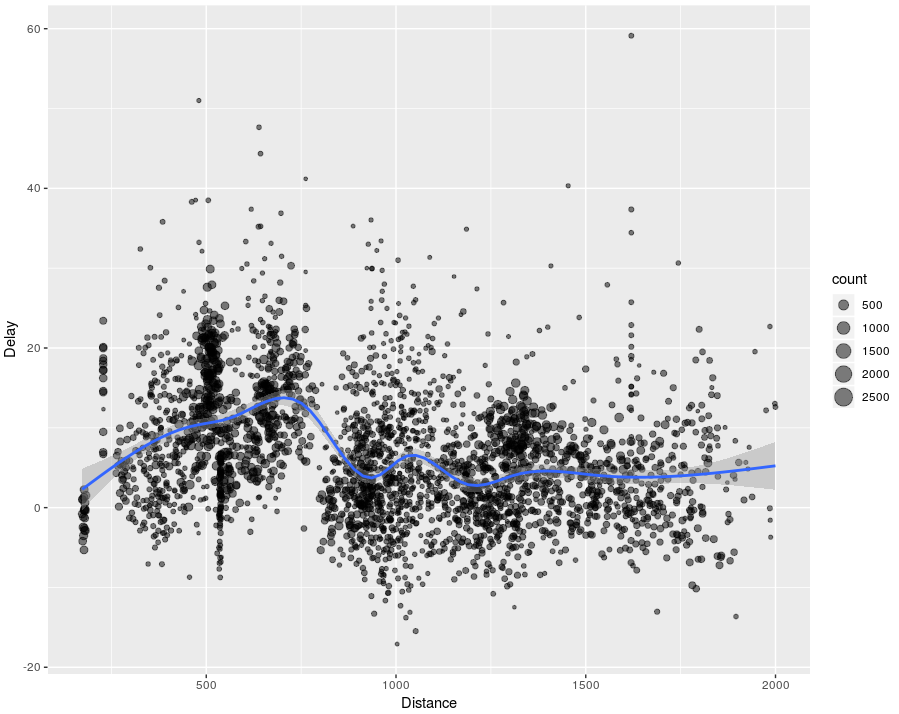#### Make New VariablesIt is often necessary to add new columns that are functions of existing columns. This is the job of the mutate() function.

The mutate() function is similar to the transform() function in base R, but has the advanatage that you can refer to columns that you’ve just created:

If you only want to keep the new variables, use transmute():

There are  set of functions common to many database tools that can be used with mutate for enhanced data transformations and insight:

FunctionDescription
lead()Copy with values shifted by N observations
lag()Copy with values lagged by N observations
dense_rank()Ranks with no gaps
Use desc() to reverse the direction
min_rank()Ranks where ties get min rank
Use desc() to reverse the direction
percent_rank()Ranks scaled to [0,1]
Use desc() to reverse the direction
row_number()Ranks where ties get first value
Use desc() to reverse the direction
ntile()Bin vector into N buckets
Use desc() to reverse the direction
between()Test if values are between a and b
cum_dist()cumulative distribution function with the Proportion of values less than or equal to the current rank
Use desc() to reverse the direction
cumall()Cumulative all
cumany()Cumulative any
cummean()Cumulative mean
cumsum()Cumulative sum
cummax()Cumulative max
cummin()Cumulative min
pmax()Element-wise max
pmin()Element-wise min

#### Combine Data Sets

Currently dplyr supports four types of mutating joins and two types of filtering joins.

Mutating joins combine variables from two data.frames:

• left_join(): return all rows from x, and all columns from a and b, as shown in the diagram below. Rows in a with no match in b will have NA values in the new columns. If there are multiple matches between a and b, all the matches are returned.
• right_join(): return all rows from b, and all columns from a and b. Rows in b with no match in a will have NA values in the new columns. If there are multiple matches between a and b, all matches are returned.
• inner_join(): return all rows from a where there are matching values in b, and all columns from a and b. If there are multiple matches between a and b, all matches are returned.
• full_join(): return all rows and all columns from both a and b. Where there are not matching values, returns NA for the one missing.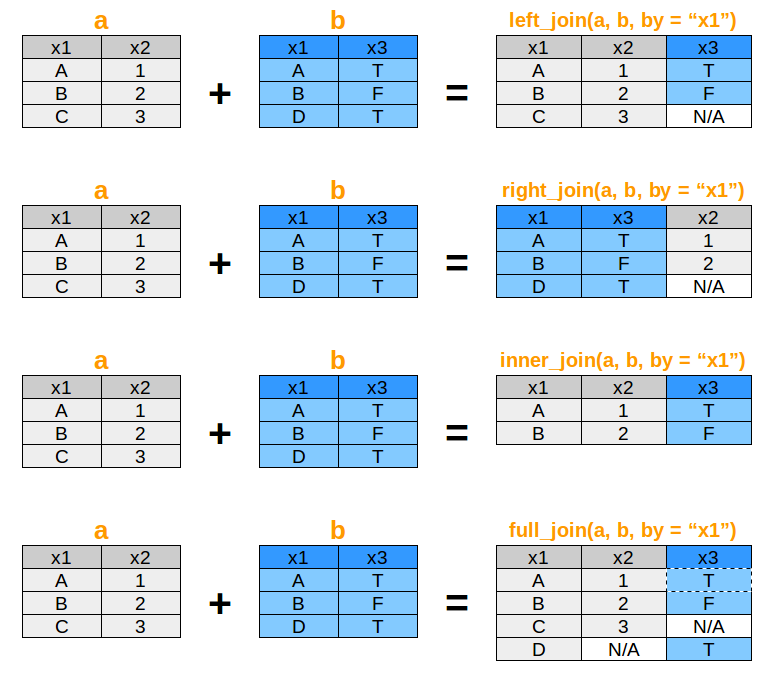Filtering joins keep cases from the left-hand data.frame:

• semi_join(): return all rows from a where there are matching values in b, keeping just columns from a, as shown below. A semi join differs from an inner join because an inner join will return one row of a for each matching row of b, where a semi join will never duplicate rows of a.
• anti_join(): return all rows from a where there are not matching values in b, keeping just columns from a.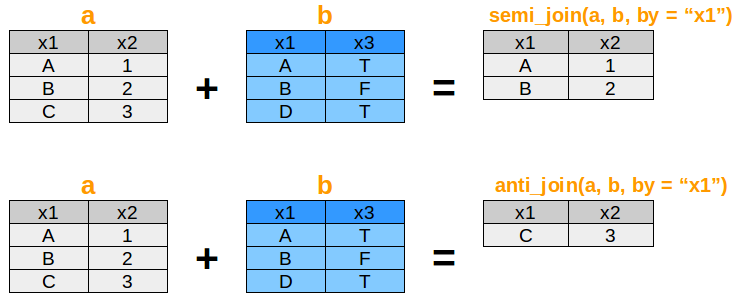1. Hadley Wickham is the creator and author of the dplyr package along with Lionel Henry Romain Francois as co-authors